### java is CALL BY VALUE (Pass by value) - NOT call by reference - With examples in java

In this core java tutorial we will learn in lots of detail that java is purely Pass by value and not Pass by reference with example, programs and diagrams. We will learn what is Call by value (Pass by value)? And how java is call by value? What is Call by reference (Pass by reference)? And what happens in Call by reference?  And how java is NOT call by reference? Programs  to demonstrate Call by value (Pass by value) in java. And, Program  to demonstrate Call by value (Pass by value) - reference to object is passed by copy in java.

Contents of page >>
• Question : What is Call by value (Pass by value)? And how java is call by value?
• Question : What is Call by reference (Pass by reference)? And what happens in Call by reference?  And how java is NOT call by reference?
• what JLS (java language specification says about JAVA being PASS BY VALUE)
• Program 1 - to demonstrate Call by value (Pass by value) in java

• Program 2 - to demonstrate Call by value (Pass by value) - reference to object is passed by copy in java
• 2.1) Let’s understand by diagram how in above program (program 2) reference to object is passed by copy in java >
• Program 3 - In continuation to program 2 - to demonstrate Call by value (Pass by value) - reference to object is passed by copy in java
• 3.1) Let’s understand by diagram how in above program (program 3) reference to object is passed by copy in java >

In java, arguments are passed by value (call by value/ Pass by value) only.
Java does not passes value by reference (call by reference/ Pass by reference).

First I will like to discuss what is difference between call by value and call by reference. And what happens in call by value and reference in java. Does object changes or not in java?
Question : What is Call by value (Pass by value)? And how java is call by value?
Answer : Call by value means Copy of an argument is passed to method. Therefore, any changes made to the argument in passed method won’t change the value of original argument.
In java when any changes are made to the argument in passed method  the value of original argument is not changed. Hence, Java is call by value.

Now, let’s move to Call by reference.
Question : What is Call by reference (Pass by reference)? And what happens in Call by reference?  And how java is NOT call by reference?
Answer : In Call by reference, reference of argument is passed to method. Therefore, any changes made to the argument in passed method will change the value of original argument as well.
In java when any changes are made to the argument in passed method  the value of original argument is not changed. Hence, Java is NOT call by reference.

Let’s see what JLS (java language specification says about JAVA being PASS BY VALUE) >
According to the JLS java is completely pass by value and not pass by reference.
When the method or constructor is invoked (§15.12), the values of the actual argument expressions initialize newly created parameter variables, each of the declared type, before execution of the body of the method or constructor. The Identifier that appears in the DeclaratorId may be used as a simple name in the body of the method or constructor to refer to the formal parameter in java.

Program 1 - to demonstrate Call by value (Pass by value) in java

In this example first we assigned some value to int i and String str, then passed them to method m(),
then we made some changes to i and str in method m()
Then we printed i and str in main method but changes were not reflected in main method. So, that shows java passed value by copy in java.

 /** Copyright (c), AnkitMittal JavaMadeSoEasy.com */ public class PassByValueExample1 {    public static void main(String[] args) {                     int i=1;  //primitive type           String str = "a"; //String object                     System.out.println("In main(), BEFORE passing by value i.e. BEFORE calling method m()");           System.out.println("i = "+i+", str = "+str);                     m(i, str); //PASS int primitive type and String object by VALUE                     System.out.println("\nIn main(), AFTER passing by value i.e. AFTER calling method m()");           System.out.println("i = "+i+", str = "+str);              }    static void m(int i, String str){           i = 2;           str = "b";           System.out.println("\nIn method m(), after making changes to parameters");           System.out.println("i = "+i+", str = "+str);              } } /*OUTPUT In main(), BEFORE passing by value i.e. BEFORE calling method m() i = 1, str = a In method m(), after making changes to parameters i = 2, str = b In main(), AFTER passing by value i.e. AFTER calling method m() i = 1, str = a */

Now, let’s analyze about call by value example - In above example Copy of an argument is passed to method m() and therefore any changes made to the argument in passed method i.e. m() didn’t change the value of original argument. in java

Program 2 - to demonstrate Call by value (Pass by value) - reference to object is passed by copy in java

Before reading below text I will like you to be careful about the terms in which reference is used. Do not mess up between reference to object and call by reference in java.

Reference to object is passed by value (i.e. copy of reference is created and passed), reference to object is not at all passed by reference in java.

Here in the below program, a is reference to object Emp a is passed by value (i.e. copy of a is created and passed), a is not at all passed by reference, it may look like that a is passed by reference but actually that doesn't happens at all copy of a is created and that is passed to method m() in java.
 class Emp{    int id;    public Emp(int id) {           this.id = id;    } } /** Copyright (c), AnkitMittal JavaMadeSoEasy.com */ public class PassByValueExample2 {    public static void main(String[] args) {           Emp a=new Emp(1);                     System.out.println("In main(), BEFORE passing a by value i.e. BEFORE calling method m()");           System.out.println("a.id = "+a.id);                     m(a); //Here a is reference to object Emp                  // a is passed by value (i.e. copy of a is created and passed),                  //a is not at all passed by reference,                  //it may look like that a is passed by reference but actually that doesn't happens at all                  //copy of a is created and that is passed to method m()           System.out.println("\nIn main(), AFTER passing a by value i.e. AFTER calling method m()");           System.out.println("a.id = "+a.id);              }    static void m(Emp b){           b.id = 2;           System.out.println("\nIn method m(), after making some changes");           System.out.println("b.id = " + b.id);    } } /*OUTPUT In main(), BEFORE passing a by value i.e. BEFORE calling method m() a.id = 1 In method m(), after making some changes b.id = 2 In main(), AFTER passing a by value i.e. AFTER calling method m() a.id = 2 */

So, we can say that reference to object is passed by copy in java.

2.1) Let’s understand by diagram how in above program (program 2) reference to object is passed by copy in java >

Emp a=new Emp(1);
A is declared as reference to object Emp.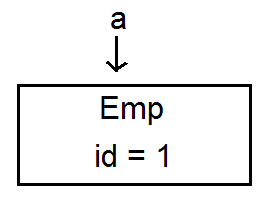static void m(Emp b)
In the method m(), Emp b is declared, b is assigned to null.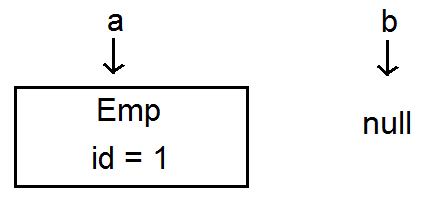m(a);
When method m is called, a is also passed to method m, but a is not directly passed to method m(), first copy of a is created and then passed to method m.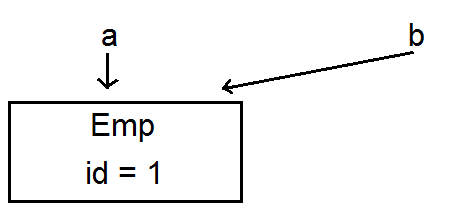b.id = 2;
b.id is assigned a new value, a and b are referring to same object so any changes made to b will be reflected in a as well.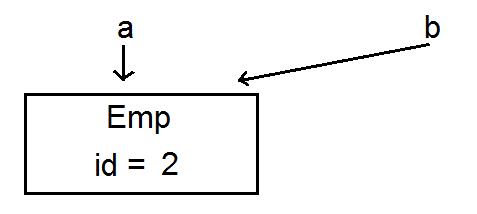We cannot change the reference in the called method i.e. the method in which reference to the object has been passed by copy, If in case reference is changed in called method, the copy of reference in the called method will start pointing to the new object but original reference will keep on pointing to old object only in java. We will understand this in next program.

Program 3 - In continuation to program 2 - to demonstrate Call by value (Pass by value) - reference to object is passed by copy in java
Reference to object is passed by value (i.e. copy of reference is created and passed), reference to object is not at all passed by reference.

Here in the below program, a is reference to object Emp, a is passed by value (i.e. copy of a is created and passed), a is not at all passed by reference, it may look like that a is passed by reference but actually that doesn't happens at all copy of a is created and that is passed to method m() in java.
We cannot change the reference b in the method m(), if in case, b is changed, b will start pointing to the new object but original reference i.e. a will keep on pointing to old object only in java.
 class Emp{    int id;    public Emp(int id) {           this.id = id;    } } /** Copyright (c), AnkitMittal JavaMadeSoEasy.com */ public class PassByValueExample3 {    public static void main(String[] args) {           Emp a=new Emp(1);                     System.out.println("In main(), BEFORE passing a by value i.e. BEFORE calling method m()");           System.out.println("a.id = "+a.id);                     m(a); //Here a is reference to object Emp                  // a is passed by value (i.e. copy of a is created and passed),                  //a is not at all passed by reference,                  //it may look like that a is passed by reference but actually that doesn't happens at all                  //copy of a is created and that is passed to method m()           System.out.println("\nIn main(), AFTER passing a by value i.e. AFTER calling method m()");           System.out.println("a.id = "+a.id);              }    static void m(Emp b){           b = new Emp(2); //Now b will start pointing to newly created object           System.out.println("\nIn method m(), after making some changes");           System.out.println("b.id = " + b.id);    } } /*OUTPUT In main(), BEFORE passing a by value i.e. BEFORE calling method m() a.id = 1 In method m(), after making some changes b.id = 2 In main(), AFTER passing a by value i.e. AFTER calling method m() a.id = 1 */

3.1) Let’s understand by diagram how in above program (program 3) reference to object is passed by copy in java >

Emp a=new Emp(1);
A is declared as reference to object Emp.static void m(Emp b)
In the method m(), Emp b is declared, b is assigned to null.m(a);
When method m is called, a is also passed to method m, but a is not directly passed to method m(), first copy of a is created and then passed to method m.b = new Emp(2);
b is assigned new Emp object. So, b will start pointing to the new object but original reference i.e. a will keep on pointing to old object only.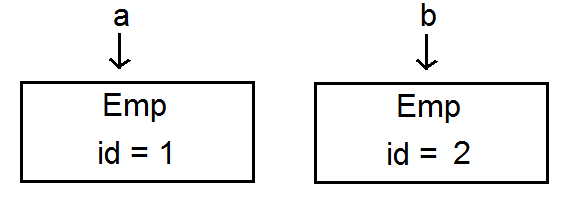So, we can say that reference to object is passed by copy in java.

So in this core java tutorial we learned in lots of detail that java is purely Pass by value and not Pass by reference with example, programs and diagrams. We learned what is Call by value (Pass by value)? And how java is call by value? What is Call by reference (Pass by reference)? And what happens in Call by reference?  And how java is NOT call by reference? Programs  to demonstrate Call by value (Pass by value) in java. And, Program  to demonstrate Call by value (Pass by value) - reference to object is passed by copy in java.